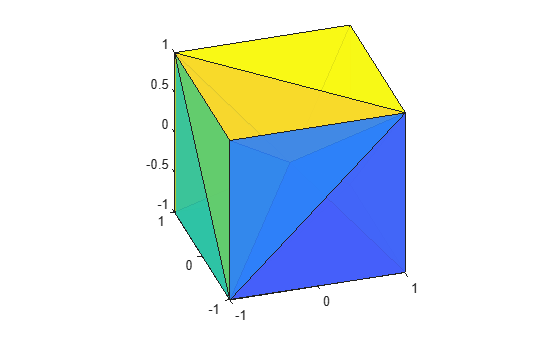Documentation

### This is machine translation

Mouseover text to see original. Click the button below to return to the English version of the page.

To view all translated materials including this page, select Country from the country navigator on the bottom of this page.

# delaunayn

N-D Delaunay triangulation

## Syntax

```T = delaunayn(X) T = delaunayn(X,options) ```

## Description

`T = delaunayn(X)` computes a set of simplices such that no data points of `X` are contained in any circumspheres of the simplices. The set of simplices forms the Delaunay triangulation. `X` is an `m`-by-`n` array representing m points in n-dimensional space. `T` is a `numt`-by-(`n+1`) array where each row contains the indices into `X` of the vertices of the corresponding simplex.

`T = delaunayn(X,options)` specifies a cell array of options. The default options are:

• `{'Qt','Qbb','Qc'}` for 2- and 3-dimensional input

• `{'Qt','Qbb','Qc','Qx'}` for 4 and higher-dimensional input

If `options` is `[]`, the default options used. If `options` is `{''}`, no options are used, not even the default.

## Visualization

Plotting the output of `delaunayn` depends of the value of `n`:

## Examples

collapse all

This example generates an n-dimensional Delaunay triangulation, where `n = 3`.

```d = [-1 1]; [x,y,z] = meshgrid(d,d,d); % A cube x = [x(:);0]; y = [y(:);0]; z = [z(:);0]; % [x,y,z] are corners of a cube plus the center. X = [x(:) y(:) z(:)]; Tes = delaunayn(X)```
```Tes = 12×4 4 3 9 1 4 9 2 1 7 9 3 1 7 5 9 1 7 9 4 3 7 8 4 9 6 2 9 1 6 9 5 1 6 4 9 2 6 4 8 9 ⋮ ```

You can use `tetramesh` to visualize the tetrahedrons that form the corresponding simplex. `camorbit` rotates the camera position to provide a meaningful view of the figure.

```tetramesh(Tes,X); camorbit(20,0)```